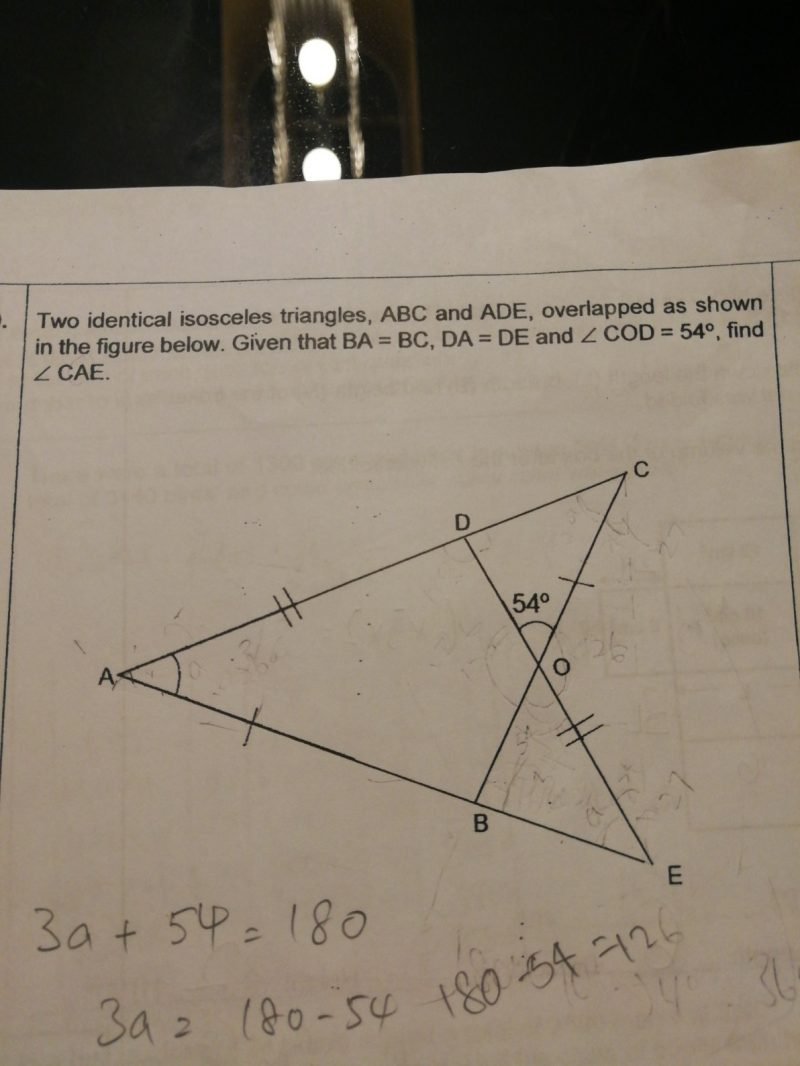# QuestionNeed help on the above question.

Thank you

Let Angle CAE = x.

Then angle ADE = 180 – 2x (isosceles triangle).

Consider triangle ODC.

180 – 2x = 54 + x (exterior angle is the sum of the opposite interior angles)

x = 42 degrees

Thank you very much! I will explain to my DD.

0 Replies 0 Likes

This question has been posted and answered before. Please check through the earlier posts for the same question.